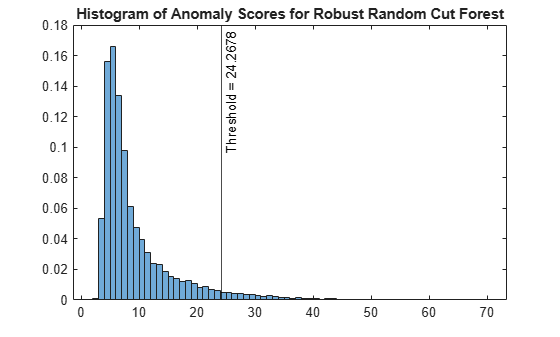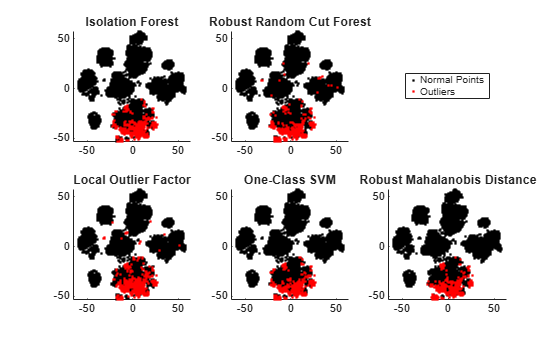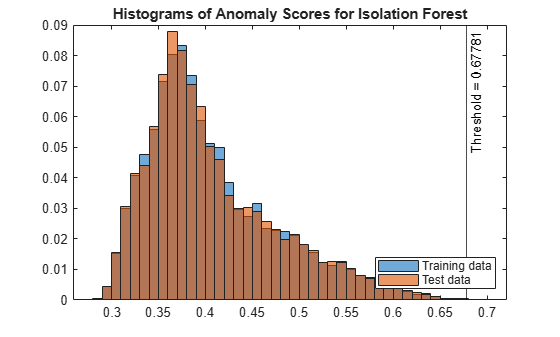## Unsupervised Anomaly Detection

This topic introduces the unsupervised anomaly detection features for multivariate sample data available in Statistics and Machine Learning Toolbox™, and describes the workflows of the features for outlier detection (detecting anomalies in training data) and novelty detection (detecting anomalies in new data with uncontaminated training data).

For unlabeled multivariate sample data, you can detect anomalies by using isolation forest, robust random cut forest, local outlier factor, one-class support vector machine (SVM), and Mahalanobis distance. These methods detect outliers either by training a model or by learning parameters. For novelty detection, you train a model or learn parameters with uncontaminated training data (data with no outliers) and detect anomalies in new data by using the trained model or learned parameters.

• Isolation forest — The Isolation Forest algorithm detects anomalies by isolating them from normal points using an ensemble of isolation trees. Detect outliers by using the `iforest` function, and detect novelties by using the object function `isanomaly`.

• Random robust cut forest — The Robust Random Cut Forest algorithm classifies a point as a normal point or an anomaly based on the changes in model complexity introduced by the point. Similar to the isolation forest algorithm, the robust random cut forest algorithm builds an ensemble of trees. The two algorithms differ in how they choose a split variable in trees and how they define anomaly scores. Detect outliers by using the `rrcforest` function, and detect novelties by using the object function `isanomaly`.

• Local outlier factor — The Local Outlier Factor (LOF) algorithm detects anomalies based on the relative density of an observation with respect to the surrounding neighborhood. Detect outliers by using the `lof` function, and detect novelties by using the object function `isanomaly`.

• One-class support vector machine (SVM) — One-class SVM, or unsupervised SVM, tries to separate data from the origin in the transformed high-dimensional predictor space. Detect outliers by using the `ocsvm` function, and detect novelties by using the object function `isanomaly`.

• Mahalanobis Distance — If sample data follows a multivariate normal distribution, then the squared Mahalanobis distances from samples to the distribution follow a chi-square distribution. Therefore, you can use the distances to detect anomalies based on the critical values of the chi-square distribution. For outlier detection, use the `robustcov` function to compute robust Mahalanobis distances. For novelty detection, you can compute Mahalanobis distances by using the `robustcov` and `pdist2` functions.

### Outlier Detection

This example illustrates the workflows of the five unsupervised anomaly detection methods (isolation forest, robust random cut forest, local outlier factor, one-class SVM, and Mahalanobis distance) for outlier detection.

Load the `humanactivity` data set, which contains the variables `feat` and `actid`. The variable `feat` contains the predictor data matrix of 60 features for 24,075 observations, and the response variable `actid` contains the activity IDs for the observations as integers. This example uses the `feat` variable for anomaly detection.

`load humanactivity`

Find the size of the variable `feat`.

`[N,D] = size(feat)`
```N = 24075 ```
```D = 60 ```

Assume that the fraction of outliers in the data is 0.05.

`contaminationFraction = 0.05;`

Isolation Forest

Detect outliers by using the `iforest` function.

Train an isolation forest model by using the `iforest` function. Specify the fraction of outliers (`ContaminationFraction`) as 0.05.

```rng("default") % For reproducibility [forest,tf_forest,s_forest] = iforest(feat, ... ContaminationFraction=contaminationFraction);```

`forest` is an `IsolationForest` object. `iforest` also returns the anomaly indicators (`tf_forest`) and anomaly scores (`s_forest`) for the data (`feat`). `iforest` determines the score threshold value (`forest.ScoreThreshold`) so that the function detects the specified fraction of observations as outliers.

Plot a histogram of the score values. Create a vertical line at the score threshold corresponding to the specified fraction.

```figure histogram(s_forest,Normalization="probability") xline(forest.ScoreThreshold,"k-", ... join(["Threshold =" forest.ScoreThreshold])) title("Histogram of Anomaly Scores for Isolation Forest")```Check the fraction of detected anomalies in the data.

`OF_forest = sum(tf_forest)/N`
```OF_forest = 0.0496 ```

The outlier fraction can be smaller than the specified fraction (0.05) when the scores have tied values at the threshold.

Robust Random Cut Forest

Detect outliers by using the `rrcforest` function.

Train a robust random cut forest model by using the `rrcforest` function. Specify the fraction of outliers (`ContaminationFraction`) as 0.05, and specify `StandardizeData` as `true` to standardize the input data.

```rng("default") % For reproducibility [rforest,tf_rforest,s_rforest] = rrcforest(feat, ... ContaminationFraction=contaminationFraction,StandardizeData=true);```

`rforest` is a `RobustRandomCutForest` object. `rrcforest` also returns the anomaly indicators (`tf_rforest`) and anomaly scores (`s_rforest`) for the data (`feat`). `rrcforest` determines the score threshold value (`rforest.ScoreThreshold`) so that the function detects the specified fraction of observations as outliers.

Plot a histogram of the score values. Create a vertical line at the score threshold corresponding to the specified fraction.

```figure histogram(s_rforest,Normalization="probability") xline(rforest.ScoreThreshold,"k-", ... join(["Threshold =" rforest.ScoreThreshold])) title("Histogram of Anomaly Scores for Robust Random Cut Forest")```Check the fraction of detected anomalies in the data.

`OF_rforest = sum(tf_rforest)/N`
```OF_rforest = 0.0500 ```

Local Outlier Factor

Detect outliers by using the `lof` function.

Train a local outlier factor model by using the `lof` function. Specify the fraction of outliers (`ContaminationFraction`) as 0.05, 500 nearest neighbors, and the Mahalanobis distance.

```[LOFObj,tf_lof,s_lof] = lof(feat, ... ContaminationFraction=contaminationFraction, ... NumNeighbors=500,Distance="mahalanobis");```

`LOFObj` is a `LocalOutlierFactor` object. `lof` also returns the anomaly indicators (`tf_lof`) and anomaly scores (`s_lof`) for the data (`feat`). `lof` determines the score threshold value (`LOFObj.ScoreThreshold`) so that the function detects the specified fraction of observations as outliers.

Plot a histogram of the score values. Create a vertical line at the score threshold corresponding to the specified fraction.

```figure histogram(s_lof,Normalization="probability") xline(LOFObj.ScoreThreshold,"k-", ... join(["Threshold =" LOFObj.ScoreThreshold])) title("Histogram of Anomaly Scores for Local Outlier Factor")```Check the fraction of detected anomalies in the data.

`OF_lof = sum(tf_lof)/N`
```OF_lof = 0.0500 ```

One-Class SVM

Detect outliers by using the `ocsvm` function.

Train a one-class SVM model by using the `ocsvm` function. Specify the fraction of outliers (`ContaminationFraction`) as 0.05. In addition, set `KernelScale` to `"auto"` to let the function select an appropriate kernel scale parameter using a heuristic procedure, and specify `StandardizeData` as `true` to standardize the input data.

```[Mdl,tf_OCSVM,s_OCSVM] = ocsvm(feat, ... ContaminationFraction=contaminationFraction, ... KernelScale="auto",StandardizeData=true);```

`Mdl` is a `OneClassSVM` object. `ocsvm` also returns the anomaly indicators (`tf_OCSVM`) and anomaly scores (`s_OCSVM`) for the data (`feat`). `ocsvm` determines the score threshold value (`Mdl.ScoreThreshold`) so that the function detects the specified fraction of observations as outliers.

Plot a histogram of the score values. Create a vertical line at the score threshold corresponding to the specified fraction.

```figure histogram(s_OCSVM,Normalization="probability") xline(Mdl.ScoreThreshold,"k-", ... join(["Threshold =" Mdl.ScoreThreshold])) title("Histogram of Anomaly Scores for One-Class SVM")```Check the fraction of detected anomalies in the data.

`OF_OCSVM = sum(tf_OCSVM)/N`
```OF_OCSVM = 0.0500 ```

Mahalanobis Distance

Use the `robustcov` function to compute robust Mahalanobis distances and robust estimates for the mean and covariance of the data.

Compute the Mahalanobis distance from `feat` to the distribution of `feat` by using the `robustcov` function. Specify the fraction of outliers (`OutlierFraction`) as 0.05. `robustcov` minimizes the covariance determinant over 95% of the observations.

```[sigma,mu,s_robustcov,tf_robustcov_default] = robustcov(feat, ... OutlierFraction=contaminationFraction);```

`robustcov` finds the robust covariance matrix estimate (`sigma`) and robust mean estimate (`mu`), which are less sensitive to outliers than the estimates from the `cov` and `mean` functions. The `robustcov` function also computes the Mahalanobis distances (`s_robustcov`) and the outlier indicators (`tf_robustcov_default`). By default, the function assumes that the data set follows a multivariate normal distribution, and identifies 2.5% of input observations as outliers based on the critical values of the chi-square distribution.

If the data set satisfies the normality assumption, then the squared Mahalanobis distance follows a chi-square distribution with `D` degrees of freedom, where `D` is the dimension of the data. In that case, you can find a new threshold by using the `chi2inv` function to detect the specified fraction of observations as outliers.

```s_robustcov_threshold = sqrt(chi2inv(1-contaminationFraction,D)); tf_robustcov = s_robustcov > s_robustcov_threshold;```

Create a distance-distance plot (DD plot) to check the multivariate normality of the data.

```figure d_classical = pdist2(feat,mean(feat),"mahalanobis"); gscatter(d_classical,s_robustcov,tf_robustcov,"kr",".x") xline(s_robustcov_threshold,"k-") yline(s_robustcov_threshold,"k-", ... join(["Threshold = " s_robustcov_threshold])); l = refline([1 0]); l.Color = "k"; xlabel("Mahalanobis Distance") ylabel("Robust Distance") legend("Normal Points","Outliers",Location="northwest") title("Distance-Distance Plot")```Zoom in the axes to see the normal points.

```xlim([0 10]) ylim([0 10])```If a data set follows a multivariate normal distribution, then data points cluster tightly around the 45 degree reference line. The DD plot indicates that the data set does not follow a multivariate normal distribution.

Because the data set does not satisfy the normality assumption, use the quantile of the distance values for the cumulative probability (`1 — contaminationFraction`) to find a threshold.

`s_robustcov_threshold = quantile(s_robustcov,1-contaminationFraction);`

Obtain the anomaly indicators for `feat` using the new threshold `s_robustcov_threshold`.

`tf_robustcov = s_robustcov > s_robustcov_threshold;`

Check the fraction of detected anomalies in the data.

`OF_robustcov = sum(tf_robustcov)/N`
```OF_robustcov = 0.0500 ```

Compare Detected Outliers

To visualize the detected outliers, reduce the data dimension by using the `tsne` function.

```rng("default") % For reproducibility T = tsne(feat,Standardize=true,Perplexity=100,Exaggeration=20);```

Plot the normal points and outliers in the reduced dimension. Compare the results of the five methods: the isolation forest algorithm, robust random cut forest algorithm, local outlier factor algorithm, one-class SVM model, and robust Mahalanobis distance from `robustcov`.

```figure tiledlayout(2,3) nexttile gscatter(T(:,1),T(:,2),tf_forest,"kr",[],[],"off") title("Isolation Forest") nexttile gscatter(T(:,1),T(:,2),tf_rforest,"kr",[],[],"off") title("Robust Random Cut Forest") nexttile(4) gscatter(T(:,1),T(:,2),tf_lof,"kr",[],[],"off") title("Local Outlier Factor") nexttile(5) gscatter(T(:,1),T(:,2),tf_OCSVM,"kr",[],[],"off") title("One-Class SVM") nexttile(6) gscatter(T(:,1),T(:,2),tf_robustcov,"kr",[],[],"off") title("Robust Mahalanobis Distance") l = legend("Normal Points","Outliers"); l.Layout.Tile = 3;```The novelties identified by the five methods are located near each other in the reduced dimension.

You can also visualize observation values using the two most important features selected by the `fsulaplacian` function.

```idx = fsulaplacian(feat); figure t = tiledlayout(2,3); nexttile gscatter(feat(:,idx(1)),feat(:,idx(2)),tf_forest,"kr",[],[],"off") title("Isolation Forest") nexttile gscatter(feat(:,idx(1)),feat(:,idx(2)),tf_rforest,"kr",[],[],"off") title("Robust Random Cut Forest") nexttile(4) gscatter(feat(:,idx(1)),feat(:,idx(2)),tf_lof,"kr",[],[],"off") title("Local Outlier Factor") nexttile(5) gscatter(feat(:,idx(1)),feat(:,idx(2)),tf_OCSVM,"kr",[],[],"off") title("One-Class SVM") nexttile(6) gscatter(feat(:,idx(1)),feat(:,idx(2)),tf_robustcov,"kr",[],[],"off") title("Mahalanobis Distance") l = legend("Normal Points","Outliers"); l.Layout.Tile = 3; xlabel(t,join(["Column" idx(1)])) ylabel(t,join(["Column" idx(2)]))```### Novelty Detection

This example illustrates the workflows of the five unsupervised anomaly detection methods (isolation forest, robust random cut forest, local outlier factor, one-class SVM, and Mahalanobis distance) for novelty detection.

Load the `humanactivity` data set, which contains the variables `feat` and `actid`. The variable `feat` contains the predictor data matrix of 60 features for 24,075 observations, and the response variable `actid` contains the activity IDs for the observations as integers. This example uses the `feat` variable for anomaly detection.

`load humanactivity`

Partition the data into training and test sets by using the `cvpartition` function. Use 50% of the observations as training data and 50% of the observations as test data for novelty detection.

```rng("default") % For reproducibility c = cvpartition(actid,Holdout=0.50); trainingIndices = training(c); % Indices for the training set testIndices = test(c); % Indices for the test set XTrain = feat(trainingIndices,:); XTest = feat(testIndices,:);```

Assume that the training data is not contaminated (no outliers).

Find the size of the training and test sets.

`[N,D] = size(XTrain)`
```N = 12038 ```
```D = 60 ```
`NTest = size(XTest,1)`
```NTest = 12037 ```

Isolation Forest

Detect novelties using the object function `isanomaly` after training an isolation forest model by using the `iforest` function.

Train an isolation forest model.

`[forest,tf_forest,s_forest] = iforest(XTrain);`

`forest` is an `IsolationForest` object. `iforest` also returns the anomaly indicators (`tf_forest`) and anomaly scores (`s_forest`) for the training data (`XTrain`). By default, `iforest` treats all training observations as normal observations, and sets the score threshold (`forest.ScoreThreshold`) to the maximum score value.

Use the trained isolation forest model and the object function `isanomaly` to find novelties in `XTest`. The `isanomaly` function identifies observations with scores above the threshold (`forest.ScoreThreshold`) as novelties.

`[tfTest_forest,sTest_forest] = isanomaly(forest,XTest);`

The `isanomaly` function returns the anomaly indicators (`tfTest_forest`) and anomaly scores (`sTest_forest`) for the test data.

Plot histograms of the score values. Create a vertical line at the score threshold.

```figure histogram(s_forest,Normalization="probability") hold on histogram(sTest_forest,Normalization="probability") xline(forest.ScoreThreshold,"k-", ... join(["Threshold =" forest.ScoreThreshold])) legend("Training data","Test data",Location="southeast") title("Histograms of Anomaly Scores for Isolation Forest") hold off```The anomaly score distribution of the test data is similar to that of the training data, so `isanomaly` detects a small number of anomalies in the test data.

Check the fraction of detected anomalies in the test data.

`NF_forest = sum(tfTest_forest)/NTest`
```NF_forest = 8.3077e-05 ```

Display the observation index of the anomalies in the test data.

`idx_forest = find(tfTest_forest)`
```idx_forest = 3422 ```

Robust Random Cut Forest

Detect novelties using the object function `isanomaly` after training a robust random cut forest model by using the `rrcforest` function.

Train a robust random cut forest model. Specify `StandardizeData` as `true` to standardize the input data.

`[rforest,tf_rforest,s_rforest] = rrcforest(XTrain,StandardizeData=true);`

`rforest` is a `RobustRandomCutForest` object. `rrcforest` also returns the anomaly indicators (`tf_rforest`) and anomaly scores (`s_rforest`) for the training data (`XTrain`). By default, `rrcforest` treats all training observations as normal observations, and sets the score threshold (`rforest.ScoreThreshold`) to the maximum score value.

Use the trained robust random cut forest model and the object function `isanomaly` to find novelties in `XTest`. The `isanomaly` function identifies observations with scores above the threshold (`rforest.ScoreThreshold`) as novelties.

`[tfTest_rforest,sTest_rforest] = isanomaly(rforest,XTest);`

The `isanomaly` function returns the anomaly indicators (`tfTest_rforest`) and anomaly scores (`sTest_rforest`) for the test data.

Plot histograms of the score values. Create a vertical line at the score threshold.

```figure histogram(s_rforest,Normalization="probability") hold on histogram(sTest_rforest,Normalization="probability") xline(rforest.ScoreThreshold,"k-", ... join(["Threshold =" rforest.ScoreThreshold])) legend("Training data","Test data",Location="southeast") title("Histograms of Anomaly Scores for Robust Random Cut Forest") hold off```The anomaly score distribution of the test data is similar to that of the training data, so `isanomaly` detects a small number of anomalies in the test data.

Check the fraction of detected anomalies in the test data.

`NF_rforest = sum(tfTest_rforest)/NTest`
```NF_rforest = 0 ```

The anomaly score distribution of the test data is similar to that of the training data, so `isanomaly` does not detect any anomalies in the test data.

Local Outlier Factor

Detect novelties using the object function `isanomaly` after training a local outlier factor model by using the `lof` function.

Train a local outlier factor model.

`[LOFObj,tf_lof,s_lof] = lof(XTrain);`

`LOFObj` is a `LocalOutlierFactor` object. `lof` returns the anomaly indicators (`tf_lof`) and anomaly scores (`s_lof`) for the training data (`XTrain`). By default, `lof` treats all training observations as normal observations, and sets the score threshold (`LOFObj.ScoreThreshold`) to the maximum score value.

Use the trained local outlier factor model and the object function `isanomaly` to find novelties in `XTest`. The `isanomaly` function identifies observations with scores above the threshold (`LOFObj.ScoreThreshold`) as novelties.

`[tfTest_lof,sTest_lof] = isanomaly(LOFObj,XTest);`

The `isanomaly` function returns the anomaly indicators (`tfTest_lof`) and anomaly scores (`sTest_lof`) for the test data.

Plot histograms of the score values. Create a vertical line at the score threshold.

```figure histogram(s_lof,Normalization="probability") hold on histogram(sTest_lof,Normalization="probability") xline(LOFObj.ScoreThreshold,"k-", ... join(["Threshold =" LOFObj.ScoreThreshold])) legend("Training data","Test data",Location="southeast") title("Histograms of Anomaly Scores for Local Outlier Factor") hold off```The anomaly score distribution of the test data is similar to that of the training data, so `isanomaly` detects a small number of anomalies in the test data.

Check the fraction of detected anomalies in the test data.

`NF_lof = sum(tfTest_lof)/NTest`
```NF_lof = 8.3077e-05 ```

Display the observation index of the anomalies in the test data.

`idx_lof = find(tfTest_lof)`
```idx_lof = 8704 ```

One-Class SVM

Detect novelties using the object function `isanomaly` after training a one-class SVM model by using the `ocsvm` function.

Train a one-class SVM model. Set `KernelScale` to `"auto"` to let the function select an appropriate kernel scale parameter using a heuristic procedure, and specify `StandardizeData` as `true` to standardize the input data.

```[Mdl,tf_OCSVM,s_OCSVM] = ocsvm(XTrain, ... KernelScale="auto",StandardizeData=true);```

`Mdl` is a `OneClassSVM` object. `ocsvm` returns the anomaly indicators (`tf_OCSVM`) and anomaly scores (`s_OCSVM`) for the training data (`XTrain`). By default, `ocsvm` treats all training observations as normal observations, and sets the score threshold (`Mdl.ScoreThreshold`) to the maximum score value.

Use the trained one-class SVM model and the object function `isanomaly` to find novelties in the test data (`XTest`). The `isanomaly` function identifies observations with scores above the threshold (`Mdl.ScoreThreshold`) as novelties.

`[tfTest_OCSVM,sTest_OCSVM] = isanomaly(Mdl,XTest);`

The `isanomaly` function returns the anomaly indicators (`tfTest_OCSVM`) and anomaly scores (`sTest_OCSVM`) for the test data.

Plot histograms of the score values. Create a vertical line at the score threshold.

```figure histogram(s_OCSVM,Normalization="probability") hold on histogram(sTest_OCSVM,Normalization="probability") xline(Mdl.ScoreThreshold,"k-", ... join(["Threshold =" Mdl.ScoreThreshold])) legend("Training data","Test data",Location="southeast") title("Histograms of Anomaly Scores for One-Class SVM") hold off```Check the fraction of detected anomalies in the test data.

`NF_OCSVM = sum(tfTest_OCSVM)/NTest`
```NF_OCSVM = 1.6615e-04 ```

Display the observation index of the anomalies in the test data.

`idx_OCSVM = find(tfTest_OCSVM)`
```idx_OCSVM = 2×1 3560 8316 ```

Mahalanobis Distance

Use the `robustcov` function to compute Mahalanobis distances of training data, and use the `pdist2` function to compute Mahalanobis distances of test data.

Compute the Mahalanobis distance from `XTrain` to the distribution of `XTrain` by using the `robustcov` function. Specify the fraction of outliers (`OutlierFraction`) as 0.

`[sigma,mu,s_mahal] = robustcov(XTrain,OutlierFraction=0);`

`robustcov` also returns the estimates of covariance matrix (`sigma`) and mean (`mu`), which you can use to compute distances of test data.

Use the maximum value of `s_mahal` as the score threshold for novelty detection.

`s_mahal_threshold = max(s_mahal);`

Compute the Mahalanobis distance from `XTest` to the distribution of `XTrain` by using the `pdist2` function.

`sTest_mahal = pdist2(XTest,mu,"mahalanobis",sigma);`

Obtain the anomaly indicators for `XTest`.

`tfTest_mahal = sTest_mahal > s_mahal_threshold;`

Plot histograms of the score values.

```figure histogram(s_mahal,Normalization="probability"); hold on histogram(sTest_mahal,Normalization="probability"); xline(s_mahal_threshold,"k-", ... join(["Threshold =" s_mahal_threshold])) legend("Training data","Test Data",Location="southeast") title("Histograms of Mahalanobis Distances") hold off```Check the fraction of detected anomalies in the test data.

`NF_mahal = sum(tfTest_mahal)/NTest`
```NF_mahal = 8.3077e-05 ```

Display the observation index of the anomalies in the test data.

`idx_mahal = find(tfTest_mahal)`
```idx_mahal = 3654 ```